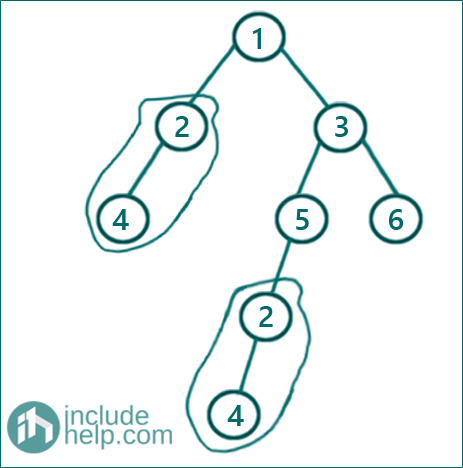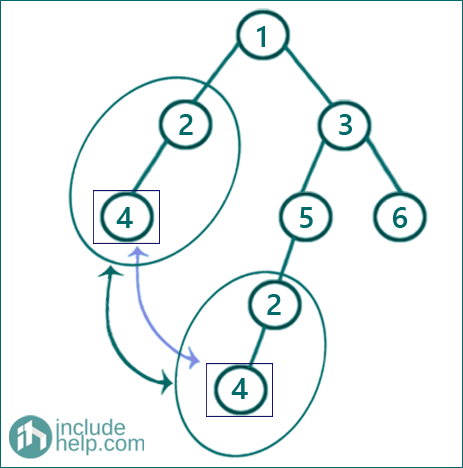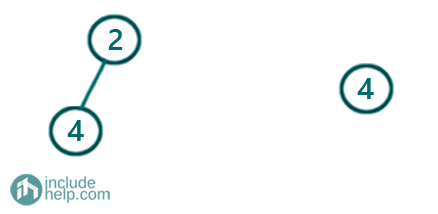# Find duplicate subtrees in a given Binary Tree

In this article, we are going to see how we can find duplicates in a binary tree using serialization?
Submitted by Radib Kar, on September 12, 2020

In this article, we are going to see how we can find duplicate subtrees in the given binary tree?

In the below example,The tree has a duplicate subtree which is,A subtree to be discovered as duplicate has to have at least two nodes. A single node subtree is not considered even if found having another occurrence. For example,The above tree doesn't have any duplicate nodes, though it has both the leaf nodes duplicate. (We are not considering it as a subtree, since it's a single node).

We can solve this problem efficiently using serialization. Serialization is a process about decoding the tree into some code which is here a string. We will follow certain serialization procedure which will help us to retrieve any duplicate subtree if exist. The idea is to serialize the entire tree recursively and while serializing storing each subtree serialization result in a hash table which helps us to find if same serialization instance is found or not. If same serialization instance is found in a hash table, we can infer that there is duplicate subtree existing the given tree. Below is the algorithm with the serialization procedure.

Prerequisites: Root of the tree, hash table hash to store serialized strings for each subtree, vector result to store each duplicate subtrees

```FUNCTION serialize (root, hash table, result)
If root is NULL
return "#";
End If
if root is leaf node
return serialized string for the root (string(root value) + ",#,#";
End If
Serialize recursively for this subtree
Serialized string is = string(root->val) + ", " +
serialization of left subtree + "," +
serialization of right subtree,
both subtree serialization is done recursively

hash[serialized string ]++;
if(the occurrence of the serialized string 2)
that means the current subtree is duplicate
Add the current subtree to the result  vector
End If
return the serialized string;
```

So after processing the vector result contains all the duplicate subtrees. Below is the C++ implementation.

```#include <bits/stdc++.h>
using namespace std;

// tree node is defined
class TreeNode {
public:
int val;
TreeNode* left;
TreeNode* right;
TreeNode(int data)
{
val = data;
left = NULL;
right = NULL;
}
};

string serialize(TreeNode* root, unordered_map<string, int>& hash, vector<TreeNode*>& result)
{
if (!root)
return "#";
if (!root->left && !root->right) {
}
string cur = to_string(root->val) + "," + serialize(root->left, hash, result) + "," + serialize(root->right, hash, result);
hash[cur]++;
//if serialized string is alreday in the hash table then it's duplicate subtree
//since we are checking hash[cur]==2 even if same3 subtree repeats for more
//than one time,we will not add to result again again
if (hash[cur] == 2) {
result.push_back(root);
}
return cur;
}

vector<TreeNode*> findDuplicateSubtrees(TreeNode* root)
{
vector<TreeNode*> result;
if (!root)
return result;
unordered_map<string, int> hash;
serialize(root, hash, result);
return result;
}

void inorder(TreeNode* root)
{

if (!root)
return;
inorder(root->left);
cout << root->val << " ";
inorder(root->right);
}

int main()
{
//building the tree like the example
TreeNode* root = new TreeNode(1);

root->left = new TreeNode(2);
root->right = new TreeNode(3);
root->left->left = new TreeNode(4);
root->right->left = new TreeNode(5);
root->right->right = new TreeNode(6);
root->right->left->left = new TreeNode(2);
root->right->left->left->left = new TreeNode(4);

vector<TreeNode*> result = findDuplicateSubtrees(root);

if (result.size() == 0)
cout << "There is no duplicate subtrees\n";
else {
cout << "inorder traversal of the duplicate subtrees are:\n";
for (auto node : result) {
inorder(node);
cout << endl;
}
}

return 0;
}
```

Output:

```inorder traversal of the duplicate subtrees are:
4 2
```

Dry Run:

Below is a few steps where we show how the recursion is working and the serialization is happening.

So,

Initially, the hash table & result vector are empty and our tree is the same one shown in the example

we call,

```serialize(root, hash, result);
--------------------------------------------------

serialize( 1, hash, result)
root is not NULL nor a leaf node
so cur= "1"+"," +  serialize( 1->left, hash, result) + serialize( 1->right, hash, result)
So first it calls to serialize( 1->left, hash, result)

--------------------------------------------------
serialize( 2, hash, result)
root is not NULL nor a leaf node
so cur= "2"+"," +  serialize( 2->left, hash, result) + serialize( 2->right, hash, result)
So first it calls to serialize( 2->left, hash, result)
--------------------------------------------------

serialize( 4, hash, result)
root is not NULL but it's  a leaf node
so it returns "4,#,#" back to the calling function  serialize( 2, hash, result)

--------------------------------------------------
serialize( 2, hash, result)
Now, It calls serialize( 2->right, hash, result) which return "#" as that's NULL
So , now cur= "2" + ","+"4,#,#" +","+"#"= "2,4,#,#,#";
Now store this in the hash table
"2,4,#,#,#"	1

And returns "2,4,#,#,#" to the calling function serialize( 1, hash, result)

--------------------------------------------------
serialize( 1, hash, result)
It now calls serialize( 1->right, hash, result)

--------------------------------------------------
serialize( 3, hash, result)
root is not NULL nor a leaf node
so cur= "3"+"," +  serialize( 3->left, hash, result) + serialize( 3->right, hash, result)
So first it calls to serialize( 3->left, hash, result)

--------------------------------------------------
serialize( 5, hash, result)
root is not NULL nor a leaf node
so cur= "5"+"," +  serialize( 5->left, hash, result) + serialize( 5->right, hash, result)
So first it calls to serialize( 5->left, hash, result)

--------------------------------------------------
serialize( 2, hash, result)
root is not NULL nor a leaf node
so cur= "2"+"," +  serialize( 2->left, hash, result) + serialize( 2->right, hash, result)
So first it calls to serialize( 2->left, hash, result)

--------------------------------------------------
serialize( 4, hash, result)
root is not NULL but it's  a leaf node
so it returns "4,#,#" back to the calling function  serialize( 2, hash, result)

--------------------------------------------------
serialize( 2, hash, result)
Now, It calls serialize( 2->right, hash, result) which return "#" as that's NULL
So , now cur= "2" + ","+"4,#,#" +","+"#"= "2,4,#,#,#";
Now store this in the hash table
"2,4,#,#,#"	2

So, here now we find the duplicate and hence add this current root( subtree) to the result vector
And returns "2,4,#,#,#" to the calling function serialize( 5, hash, result)

--------------------------------------------------
serialize(5, hash, result)
It now calls Serialize(5->right, hash, result) which return "#" since that's a NULL node
So now cur is "5"+"," +"2,4,#,#,#"+","+"#"="5, 2,4,#,#,#,#"
"2,4,#,#,#"	2
"5, 2,4,#,#,#,#"	1
```

Now this return "5,2,4,#,#,#,#" back to the calling function  serialize(3, hash, result).

I am discarding the dry run as we have already spotted the duplicate and we know there's no more duplicate in the binary tree, but I recommend you to complete it for better visualization.

N.B: If the problem statement allows single node subtree duplicate too, then simply remove the second base case where I have checked for the leaf node so that those nodes also store its serialization in the hash table.

If we allow single node subtree duplicate also the same example will have two duplicates like below:And the duplicates are:Below is the implementation for the changed constraint:

```#include <bits/stdc++.h>
using namespace std;

// tree node is defined
class TreeNode {
public:
int val;
TreeNode* left;
TreeNode* right;
TreeNode(int data)
{
val = data;
left = NULL;
right = NULL;
}
};

string serialize(TreeNode* root, unordered_map<string, int>& hash, vector<TreeNode*>& result)
{
if (!root)
return "#";

string cur = to_string(root->val) + "," + serialize(root->left, hash, result) + "," + serialize(root->right, hash, result);
hash[cur]++;

//if serialized string is alreday in the hash table then it's duplicate subtree
//since we are checking hash[cur]==2 even if same3 subtree repeats for more
//than one time,we will not add to result again again
if (hash[cur] == 2) {
result.push_back(root);
}
return cur;
}

vector<TreeNode*> findDuplicateSubtrees(TreeNode* root)
{
vector<TreeNode*> result;
if (!root)
return result;
unordered_map<string, int> hash;
serialize(root, hash, result);
return result;
}

void inorder(TreeNode* root)
{

if (!root)
return;
inorder(root->left);
cout << root->val << " ";
inorder(root->right);
}

int main()
{
//building the tree like the example
TreeNode* root = new TreeNode(1);
root->left = new TreeNode(2);
root->right = new TreeNode(3);
root->left->left = new TreeNode(4);
root->right->left = new TreeNode(5);
root->right->right = new TreeNode(6);
root->right->left->left = new TreeNode(2);
root->right->left->left->left = new TreeNode(4);

vector<TreeNode*> result = findDuplicateSubtrees(root);
if (result.size() == 0)
cout << "There is no duplicate subtrees\n";
else {
cout << "inorder traversal of the dupliacate subtrees are:\n";
for (auto node : result) {
inorder(node);
cout << endl;
}
}
return 0;
}
```

Output:

```inorder traversal of the dupliacate subtrees are:
4
4 2
```

What's New

Top Interview Coding Problems/Challenges!

Languages: » C » C++ » C++ STL » Java » Data Structure » C#.Net » Android » Kotlin » SQL
Web Technologies: » PHP » Python » JavaScript » CSS » Ajax » Node.js » Web programming/HTML
Solved programs: » C » C++ » DS » Java » C#
Aptitude que. & ans.: » C » C++ » Java » DBMS
Interview que. & ans.: » C » Embedded C » Java » SEO » HR
CS Subjects: » CS Basics » O.S. » Networks » DBMS » Embedded Systems » Cloud Computing
» Machine learning » CS Organizations » Linux » DOS
More: » Articles » Puzzles » News/Updates